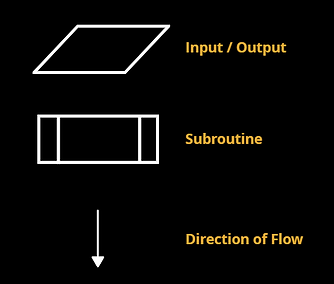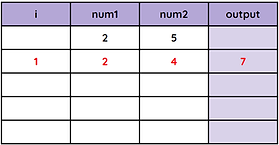top of page

## What is an algorithm?

An algorithm is a set of instructions, presented in a logical sequence.

In an exam you may be asked to read and understand an algorithm that has been written. To prove your understanding you may be asked to respond by actions such as listing the outputs of the algorithm, correcting errors or identifying an error within it.

Programmers create algorithm designs as a method of planning a program before writing any code. This helps them to consider the potential problems of the program and makes it easier to start creating source code.

There are two main methods of defining algorithms are pseudocode and flowcharts. In exams, OCR will display algorithms in their own 'OCR Exam Reference Language'.

## OCR Exam Reference Language

The OCR exams require specific questions to be written either in OCR Exam Reference Language (shown below) or a high-level programming language such as Python.

Basic Commands

Annotation

//Comments are written using two slashes

Assignment

name = "Harold"

age = 49

Constants and Global Variables

constant tax = 15

Input / Output

print("Transaction Complete")

Casting

str(29)

int("102")

float(30)

bool("False")

Random Number

number = random(1,100)

Selection

Selection (if - then - else)

if firstname == "Steven" then​

print("Hello" + firstname)

elif firstname == "Steve" then

else

print("Who are you?")

end if

Selection (case select)

switch day:

case “Sat”:

print(“It is Saturday”)

case “Sun”:

print(“It is Sunday”)

default:

print(“It is a Weekday”)

endswitch

Iteration

Iteration (for loop)

for i = 1 to 10 step 1

input item

next i

Iteration (while loop)

while firstname != "Steven"

firstname = input("Try again:")

endwhile

Iteration (do while loop)

do

firstname = input("Guess name:")

until firstname == "Steven"

String Handling

Length of a String

word = "dictionary"

print(word.length)                 outputs 10

Substrings

word = "dinosaurs"

print(word.substring(2,3))   outputs nos

print(word.left(3))                  outputs din

print(word.right(4))               outputs aurs

Concatenation

name = "Penelope"

surname = "Sunflower"

print(name + surname)

String Cases

phrase = "The Cat Sat On The Mat"

print(phrase.lower)

print(phrase.upper)

ASCII Conversion

ASC("C")            returns 67

CHR(100)          returns "d"

File Handling

file1 = open("Customers.txt")

while NOT file1.endOfFile()

endwhile

file1.close()

File Handling - Writing to a (New) File

newFile("paint.txt")

file2 = open("paint.txt")

paint = input("Enter a paint colour:")

file.writeLine(paint)

file2.close()

Arrays

Declare Array

array names

array names = "Ella", "Sam", "Ali"

Declare 2D Array

array grid[4,5]

Assign Values

names = "Samantha"

grid[1,3] = "X"

## Flowcharts

A flowchart can be used to visually represent an algorithm.

It is more likely you will need to be able to interpret a flowchart rather than draw one.

The flowchart symbols are:## Algorithm Examples

Below are two different methods for representing the same algorithm - a program to encourage people to buy items cheaply at a supermarket.

The program allows the price of items in a supermarket to be entered until the total reaches 100. The total price and the number of items entered are tracked as the program loops. Once the total reaches 100 or more, an if statement checks how many items have been entered and a different message is printed if there are 20 or more items, 30 or more items or less than 20 items.

Pseudocode

//This is a program to see how many items you can buy in a supermarket
before you spend over £100}

total = 0

itemsentered = 0

while total < 100

itemprice = input("enter the price of the next item")

total = total + itemprice

itemsentered = itemsentered + 1

endwhile

if itemsentered >= 20 then

print("You are on your way to saving money.")

elif itemsentered => 30 then

print("You're a real money saver.")

else

print("Look for better deals next time.")

endifFlowchart

In an exam you may be asked to read an algorithm and prove your understanding, most commonly by listing the outputs.

Start from the first line and follow the program line by line, recording the value of variables as you go.

When you encounter a for loop, repeat the indented code as many times as stated in the range.

Example Algorithm:

procedure NewProgram()

maxvalue = input()

for i = 1 to maxvalue

output (i * i)

???????

print("program finished")

endprocedure

Example Questions:

1. List the outputs produced by the algorithm if the 'maxvalue' input is 5.

2. State the code that has been replaced by '???????' and what the code's purpose is.

1.

Outputs:

1

4

9

16

25

program finished

2.

Missing Code: next i

Purpose: Moves the loop to the next iteration.

## Trace Tables

Trace tables are used to track the value of variables as a program is run.

They can be used to manually track the values in order to investigate why the program isn't working as intended.

Each row in the trace table represents another iteration. Each column stores the value of a variable as it changes.

See below how the trace table is updated for the simple algorithm on the left.

num1 = 2

num2 = 5

for i = 1 to 3

output (num1 + num2)

num2 = num2 - 1

next i

print("complete")For most algorithms, not every variable will be updated in each iteration.

Values may not be entered in the order of the trace table either. For example, each iteration outputs num1 + num2 and then decreases the value of num2 by 1.## Questo's Questions

1.2 - Designing Algorithms:

1. What is the definition of an algorithm? Name two ways an algorithm can be designed. 

2. Using a high-level programming language such as Python, or the OCR Exam Reference Language, write an algorithm that inputs 6 decimal numbers and outputs the total, largest, smallest and average values. 

For example, entering 3.1, 5.3, 2.3, 5.4, 2.9 and 4.4 would output 23.3 (total), 5.4 (largest), 2.3 (smallest) and 3.9 (average).

3. Draw and label the flowchart symbols. 

4. Create a trace table for the NewProgram() algorithm in the Reading Algorithms section on this page. 

bottom of page